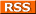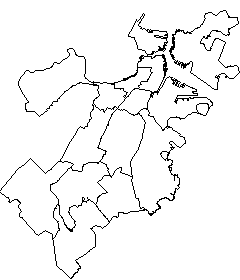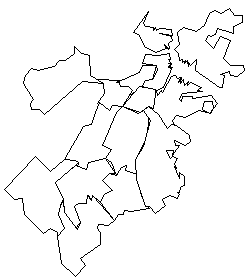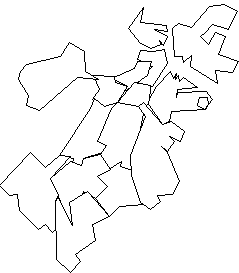Comments Rss
PostGIS Simplify

# Simplify: Reduce the weight of a geometry

Simplify reduces the complexity and weight of a geometry using the Douglas-Peucker algorithm. It in general reduces the number of vertices in a polygon, multipolygon, line, or multi-line geometry. It takes 2 arguments, the geometry and the tolerance. To demonstrate we will apply the simplify function on Boston neighborhoods that are in SRID 2249 (NAD83 / Massachusetts Mainland (ftUS)) and compare the sizes of the shape files if we were to dump these out as ESRI shape files. We will also show pictorially how simplify changes the geometries.

 Original: Size of Shp - 95 kb ```SELECT the_geom FROM neighborhoods```Simplify 500: Size of Shp - 7 kb```SELECT simplify(the_geom,500) as simpgeom FROM neighborhoods```Simplify 1000: Size of Shp - 5 kb```SELECT simplify(the_geom,1000) as simpgeom FROM neighborhoods```Note: When simplifying longlat data, you often get very strange results with simplify. It is best to first transform data from longlat to some other coordinate system, then simplify and then transform back to longlat. So something like ``` SELECT transform(simplify(transform(the_geom, 2249), 500),4326) from neighborhoods ```